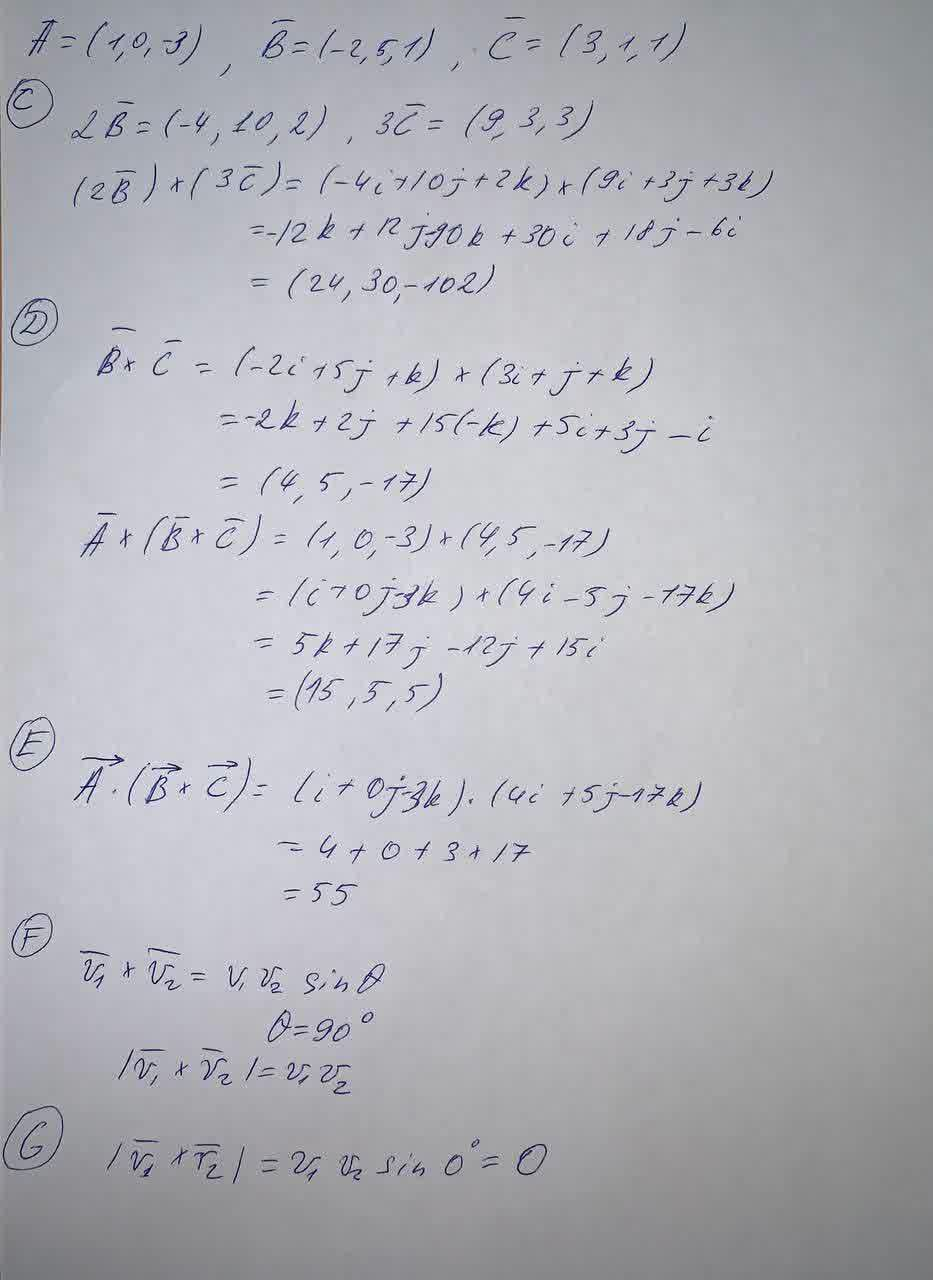Vector Cross ProductLet vectors A=(1,0,-3), B =(-2,5,1), and C =(3,1,1).EunoR 2021-05-27 Answered

Vector Cross Product
Let vectors $$A=(1,0,-3), B =(-2,5,1),\ and\ C =(3,1,1)$$. Calculate the following, expressing your answers as ordered triples (three comma-separated numbers).
(C) $$(2\bar B)(3\bar C)$$
(D) $$(\bar B)(\bar C)$$
(E) $$\overrightarrow A(\overrightarrow B \times \overrightarrow C)$$
(F)If $$\bar v_1 \text{ and } \bar v_2$$ are perpendicular, $$|\bar v_1 \times \bar v_2|$$
(G) If $$\bar v_1 \text{ and } \bar v_2$$ are parallel, $$|\bar v_1 \times \bar v_2|$$

• Questions are typically answered in as fast as 30 minutes

Solve your problem for the price of one coffee

• Math expert for every subject
• Pay only if we can solve itau4gsf